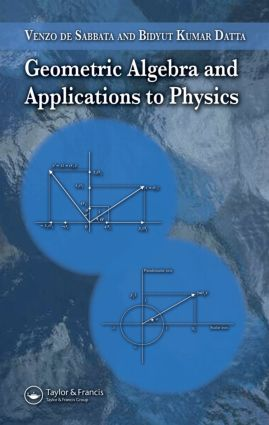# Geometric Algebra and Applications to Physics

## 1st Edition

CRC Press

184 pages | 15 B/W Illus.

##### Purchasing Options:\$ = USD
Hardback: 9781584887720
pub: 2006-12-07
Currently out of stock
\$140.00
x
eBook (VitalSource) : 9780429150333
pub: 2006-12-07
from \$28.98

FREE Standard Shipping!

### Description

Bringing geometric algebra to the mainstream of physics pedagogy, Geometric Algebra and Applications to Physics not only presents geometric algebra as a discipline within mathematical physics, but the book also shows how geometric algebra can be applied to numerous fundamental problems in physics, especially in experimental situations.

This reference begins with several chapters that present the mathematical fundamentals of geometric algebra. It introduces the essential features of postulates and their underlying framework; bivectors, multivectors, and their operators; spinor and Lorentz rotations; and Clifford algebra. The book also extends some of these topics into three dimensions. Subsequent chapters apply these fundamentals to various common physical scenarios. The authors show how Maxwell's equations can be expressed and manipulated via space-time algebra and how geometric algebra reveals electromagnetic waves' states of polarization. In addition, they connect geometric algebra and quantum theory, discussing the Dirac equation, wave functions, and fiber bundles. The final chapter focuses on the application of geometric algebra to problems of the quantization of gravity.

By covering the powerful methodology of applying geometric algebra to all branches of physics, this book provides a pioneering text for undergraduate and graduate students as well as a useful reference for researchers in the field.

### Reviews

"The book is written for physicists with at least two years’ study of university physics, but Part I develops Clifford algebra from a standing start and is suitable for new undergraduates. I hope it finds a market among them as Clifford’s language spreads. Those who persist will find that Part II is a treat in store."

– Dr. A.J.M. Garrett, Scitext, in Contemporary Physics, August 2007, Vol. 48, No. 4

THE BASIS FOR GEOMETRIC ALGEBRA

Introduction

Genesis of Geometric Algebra

Mathematical Elements of Geometric Algebra

Geometric Algebra as a Symbolic System

Geometric Algebra as an Axiomatic System (Axiom A)

Some Essential Formulas and Definitions

MULTIVECTORS

Geometric Product of Two Bivectors A and B

Operation of Reversion

Magnitude of a Multivector

Directions and Projections

Angles and Exponential Functions (as Operators)

Exponential Functions of Multivectors

EUCLIDEAN PLANE

The Algebra of Euclidean Plane

Geometric Interpretation of a Bivector of Euclidean Plane

Spinor i-Plane

Distinction between Vector and Spinor Planes

The Geometric Algebra of a Plane

THE PSEUDOSCALAR AND IMAGINARY UNIT

The Geometric Algebra of Euclidean 3-Space

Complex Conjugation

Appendix: Some Important Results

REAL DIRAC ALGEBRA

Geometric Significance of the Dirac Matrices ?µ

Geometric Algebra of Space-Time

Conjugations

Lorentz Rotations

Spinor Theory of Rotations in Three-Dimensional Euclidean Space

SPINOR AND QUATERNION ALGEBRA

Spinor Algebra: Quaternion Algebra

Vector Algebra

Clifford Algebra: Grand Synthesis of Algebra of Grassmann and Hamilton and the Geometric Algebra of Hestenes

MAXWELL EQUATIONS

Maxwell Equations in Minkowski Space-Time

Maxwell Equations in Riemann Sace-Time (V4 Manifold)

Maxwell Equations in Riemann-Cartan Space-Time (U4 Manifold)

Maxwell Equations in Terms of Space-Time Algebra (STA)

ELECTROMAGNETIC FIELD IN SPACE AND TIME (POLARIZATION OF ELECTROMAGNETIC WAVES)

Electromagnetic (EM) Waves and Geometric Algebra

Polarization of Electromagnetic Waves

Quaternion Form of Maxwell Equations from the Spinor Form of STA

Maxwell Equations in Vector Algebra from the Quaternion (Spinor) Formalism

Majorana-Weyl Equations from the Quaternion (Spinor) Formalism of Maxwell Equations

Appendix A: Complex Numbers in Electrodynamics

Appendix B: Plane-Wave Solutions to Maxwell Equations-Polarization of EM Waves

GENERAL OBSERVATIONS AND GENERATORS OF ROTATIONS (NEUTRON INTERFEROMETER EXPERIMENT)

Review of Space-Time Algebra (STA)

The Dirac Equation without Complex Numbers

Observables and the Wave Function

Generators of Rotations in Space-Time: Intrinsic Spin

Fiber Bundles and Quantum Theory vis-à-vis the Geometric Algebra Approach

Fiber Bundle Picture of the Neutron Interferometer Experiment

Charge Conjugation

Appendix

QUANTUM GRAVITY IN REAL SPACE-TIME (COMMUTATORS AND ANTICOMMUTATORS)

Quantum Gravity and Geometric Algebra

Quantum Gravity and Torsion

Quantum Gravity in Real Space-Time

Spin Fluctuations

Some Remarks and Conclusions

Appendix: Commutator and Anticommutator

INDEX

References appear at the end of each chapter.

### Subject Categories

##### BISAC Subject Codes/Headings:
MAT003000
MATHEMATICS / Applied
SCI040000
SCIENCE / Mathematical Physics